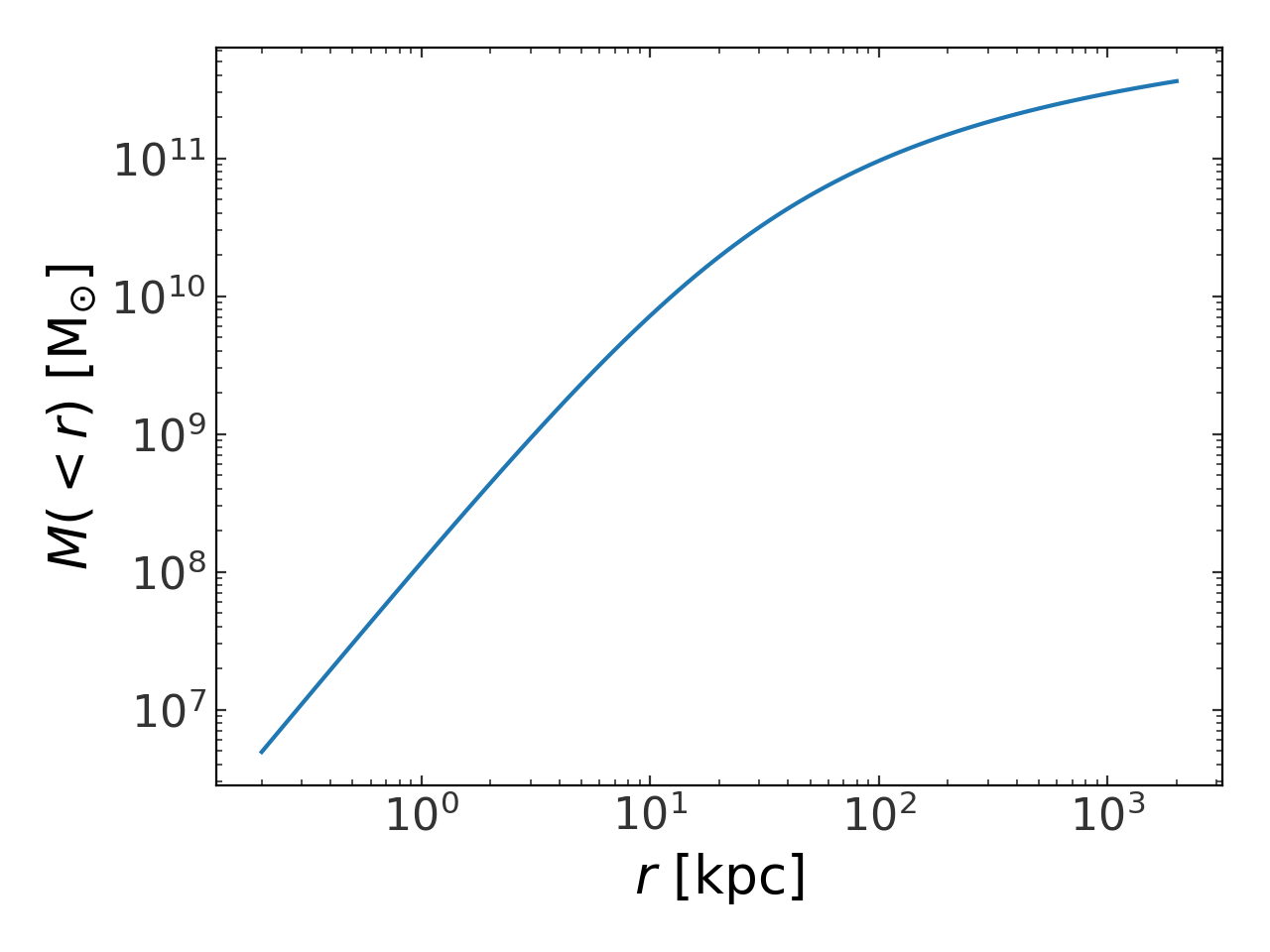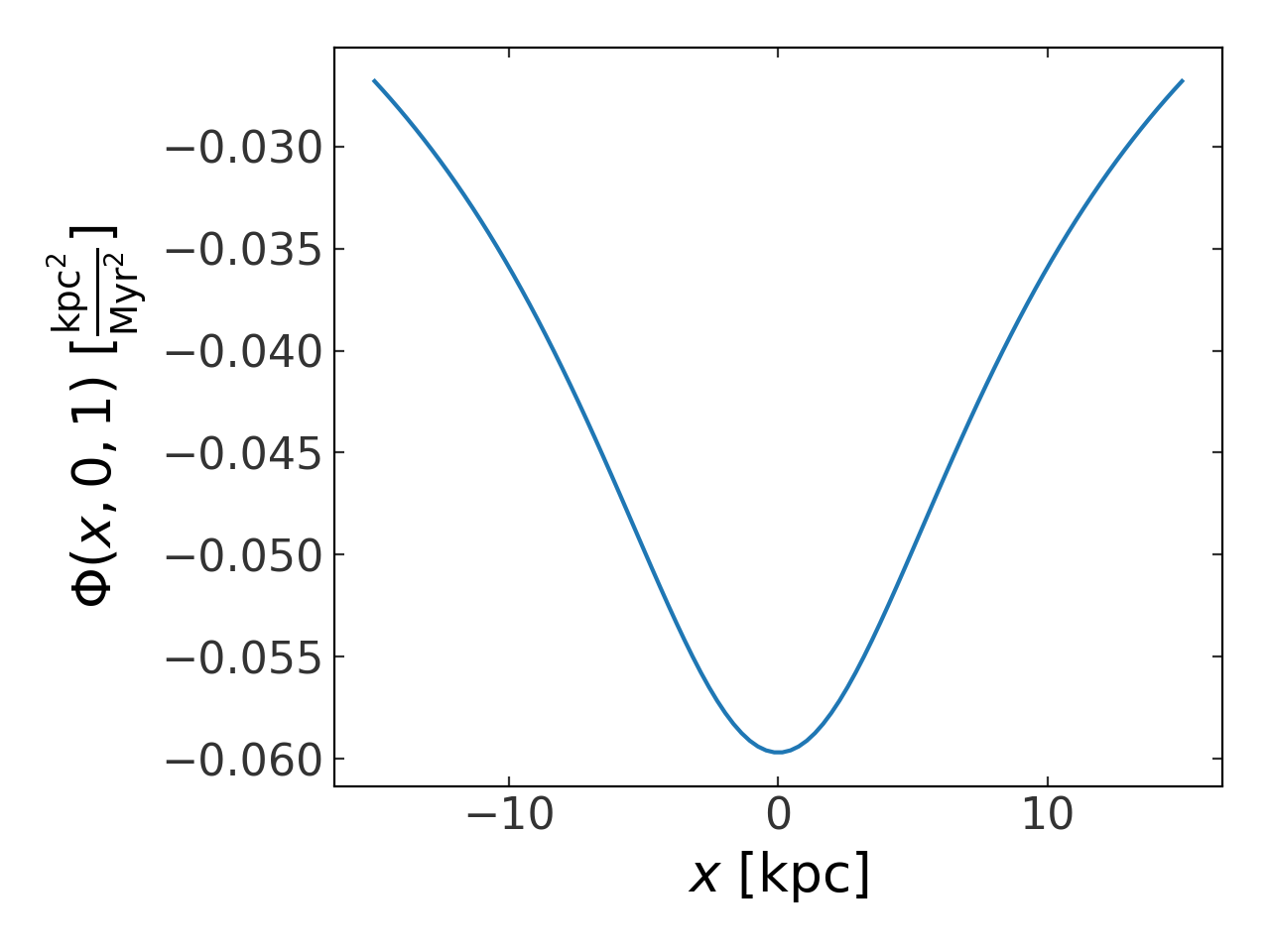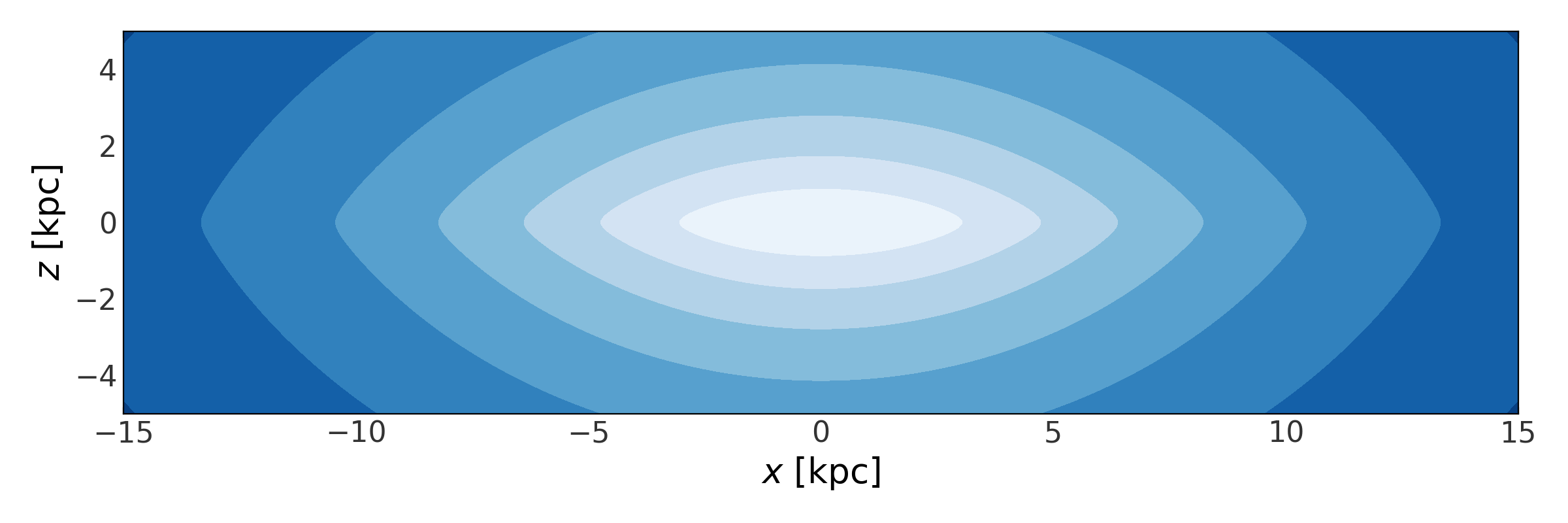# Gravitational potentials (gala.potential)¶

## Introduction¶

This subpackage provides a number of classes for working with parametric models of gravitational potentials. There are a number of built-in potentials implemented in C and Cython for speed and there are base classes that allow for easy creation of new custom potential classes in pure-Python. The potential objects have methods for computing, for example, the potential energy, gradient, density, or mass profiles. These are particularly useful in combination with the integrate and dynamics subpackages.

Also defined in this subpackage are a set of reference frames which can be used for numerical integration of orbits in non-static reference frames. See the page on Hamiltonian objects and reference frames for more information. Potential objects can be combined with a reference frame and stored in a Hamiltonian object that provides an easy interface to numerical orbit integration.

For code blocks below and any pages linked below, I assume the following imports have already been excuted:

>>> import astropy.units as u
>>> import matplotlib.pyplot as plt
>>> import numpy as np
>>> import gala.potential as gp
>>> from gala.units import galactic, solarsystem, dimensionless


## Getting started: built-in methods of potential classes¶

The built-in potentials are all initialized by passing in keyword argument parameter values as Quantity objects or as numeric values in a specified unit system. To see what parameters are available for a given potential, check the documentation for the individual classes below. You must also specify a UnitSystem when initializing a potential. A unit system is a set of non-reducible units that define (at minimum) the length, mass, time, and angle units. A few common unit systems are built in to the package (e.g., galactic, solarsystem, dimensionless).

All of the built-in potential objects have defined methods to evaluate the potential energy and the gradient/acceleration at a given position(s). For example, here we will create a potential object for a 2D point mass located at the origin with unit mass:

>>> ptmass = gp.KeplerPotential(m=1.*u.Msun, units=solarsystem)
>>> ptmass


If you pass in parameters with different units, they will be converted to the specified unit system:

>>> gp.KeplerPotential(m=1047.6115*u.Mjup, units=solarsystem)


If no units are specified for a parameter, it is assumed to already be consistent with the UnitSystem passed in:

>>> gp.KeplerPotential(m=1., units=solarsystem)


The potential classes work well with the astropy.units framework, but to ignore units you can use the DimensionlessUnitSystem or pass None as the unit system:

>>> gp.KeplerPotential(m=1., units=None)
<KeplerPotential: m=1.00 (dimensionless)>


With an instantiated potential object, we can evaluate the potential energy at some position or a set of positions. Here, we’ll evaluate a 3D point mass potential at the 3D position (1,-1,0):

>>> ptmass.energy([1.,-1.,0.]*u.au)
<Quantity [-27.91440236] AU2 / yr2>


These functions also accept both Quantity objects or plain ndarray-like objects (in which case the position is assumed to be in the unit system of the potential):

>>> ptmass.energy([1.,-1.,0.])
<Quantity [-27.91440236] AU2 / yr2>


This also works for multiple positions by passing in a 2D position (but see Conventions for a description of the interpretation of different axes):

>>> pos = np.array([[1.,-1.,0],
...                 [2.,3.,0]]).T
>>> ptmass.energy(pos*u.au)
<Quantity [-27.91440236, -10.94892941] AU2 / yr2>


We may also compute the gradient or acceleration:

>>> ptmass.gradient([1.,-1.,0]*u.au)
<Quantity [[ 13.95720118],
[-13.95720118],
[  0.        ]] AU / yr2>
>>> ptmass.acceleration([1.,-1.,0]*u.au)
<Quantity [[-13.95720118],
[ 13.95720118],
[ -0.        ]] AU / yr2>


Some of the potential objects also have methods implemented for computing the corresponding mass density and the Hessian of the potential (matrix of 2nd derivatives) at given locations. For example:

>>> pot = gp.HernquistPotential(m=1E9*u.Msun, c=1.*u.kpc, units=galactic)
>>> pot.density([1.,-1.,0]*u.kpc)
<Quantity [7997938.82200887] solMass / kpc3>
>>> pot.hessian([1.,-1.,0]*u.kpc)
<Quantity [[[ -4.68318131e-05],
[  5.92743432e-04],
[  0.00000000e+00]],

[[  5.92743432e-04],
[ -4.68318131e-05],
[  0.00000000e+00]],

[[  0.00000000e+00],
[  0.00000000e+00],
[  5.45911619e-04]]] 1 / Myr2>


Another useful method is mass_enclosed(), which numerically estimates the mass enclosed within a spherical shell defined by the specified position. This numerically estimates $$\frac{d \Phi}{d r}$$ along the vector pointing at the specified position and estimates the enclosed mass simply as $$M(<r)\approx\frac{r^2}{G} \frac{d \Phi}{d r}$$. This function can be used to compute, for example, a mass profile:

>>> pot = gp.NFWPotential(m=1E11*u.Msun, r_s=20.*u.kpc, units=galactic)
>>> pos = np.zeros((3,100)) * u.kpc
>>> pos = np.logspace(np.log10(20./100.), np.log10(20*100.), pos.shape) * u.kpc
>>> m_profile = pot.mass_enclosed(pos)
>>> plt.loglog(pos, m_profile, marker='')
>>> plt.xlabel("$r$ [{}]".format(pos.unit.to_string(format='latex')))
>>> plt.ylabel("$M(<r)$ [{}]".format(m_profile.unit.to_string(format='latex')))## Plotting isopotentials¶

Potential objects also provide more specialized methods such as plot_contours(), which is a fast way to plot either 1D slices or 2D contour plots of isopotentials. To plot a 1D slice over the dimension of interest, pass in a grid of values for that dimension and numerical values for the others. For example, to make a 1D plot of the potential value as a function of $$x$$ position at $$y=0, z=1$$:

>>> p = gp.MiyamotoNagaiPotential(m=1E11, a=6.5, b=0.27, units=galactic)
>>> fig, ax = plt.subplots(1,1)
>>> p.plot_contours(grid=(np.linspace(-15,15,100), 0., 1.), marker='', ax=ax)
>>> E_unit = p.units['energy'] / p.units['mass']
>>> ax.set_xlabel("$x$ [{}]".format(p.units['length'].to_string(format='latex')))
>>> ax.set_ylabel("$\Phi(x,0,1)$ [{}]".format(E_unit.to_string(format='latex')))To instead make a 2D contour plot over $$x$$ and $$z$$ along with $$y=0$$, pass in a 1D grid of values for $$x$$ and a 1D grid of values for $$z$$ (the meshgridding is taken care of internally). Here, we choose to draw on a pre-defined matplotlib axes object so we can set the labels and aspect ratio of the plot:

>>> fig,ax = plt.subplots(1, 1, figsize=(12,4))
>>> x = np.linspace(-15,15,100)
>>> z = np.linspace(-5,5,100)
>>> p.plot_contours(grid=(x, 1., z), ax=ax)
>>> ax.set_xlabel("$x$ [kpc]")
>>> ax.set_ylabel("$z$ [kpc]")Potential objects can be pickled and can therefore be stored for later use. However, pickles are saved as binary files. It may be useful to save to or load from text-based specifications of Potential objects. This can be done with gala.potential.save() and gala.potential.load(), or with the save() and method:

>>> from gala.potential import load
>>> pot = gp.NFWPotential(m=6E11*u.Msun, r_s=20.*u.kpc,
...                       units=galactic)
>>> pot.save("potential.yml")


## Using gala.potential¶

More details are provided in the linked pages below:

## API¶

### gala.potential.potential Package¶

#### Functions¶

 from_equation(expr, vars, pars[, name, hessian]) Create a potential class from an expression for the potential. load(f[, module]) Read a potential specification file and return a PotentialBase object instantiated with parameters specified in the spec file. save(potential, f) Write a PotentialBase object out to a text (YAML) file.

#### Classes¶

 BovyMWPotential2014([units, disk, halo, bulge]) An implementation of the MWPotential2014 from galpy and described in Bovy (2015). CCompositePotential CPotentialBase A baseclass for defining gravitational potentials implemented in C. CompositePotential(*args, **kwargs) A potential composed of several distinct components. HarmonicOscillatorPotential(omega[, units]) Represents an N-dimensional harmonic oscillator. HenonHeilesPotential([units, origin, R]) The Hénon-Heiles potential. HernquistPotential(m, c[, units, origin, R]) Hernquist potential for a spheroid. IsochronePotential(m, b[, units, origin, R]) The Isochrone potential. JaffePotential(m, c[, units, origin, R]) Jaffe potential for a spheroid. KeplerPotential(m[, units, origin, R]) The Kepler potential for a point mass. KuzminPotential(m, a, units) The Kuzmin flattened disk potential. LM10Potential([units, disk, bulge, halo]) The Galactic potential used by Law and Majewski (2010) to represent the Milky Way as a three-component sum of disk, bulge, and halo. LeeSutoTriaxialNFWPotential(v_c, r_s, a, b, c) Approximation of a Triaxial NFW Potential with the flattening in the density, not the potential. LogarithmicPotential(v_c, r_h, q1, q2, q3[, …]) Triaxial logarithmic potential. LongMuraliBarPotential(m, a, b, c[, alpha, …]) A simple, triaxial model for a galaxy bar. MilkyWayPotential([units, disk, halo, …]) A simple mass-model for the Milky Way consisting of a spherical nucleus and bulge, a Miyamoto-Nagai disk, and a spherical NFW dark matter halo. MiyamotoNagaiPotential(m, a, b[, units, …]) Miyamoto-Nagai potential for a flattened mass distribution. NFWPotential(m, r_s[, a, b, c, units, origin, R]) General Navarro-Frenk-White potential. NullPotential([units, origin, R]) A null potential with 0 mass. PlummerPotential(m, b[, units, origin, R]) Plummer potential for a spheroid. PotentialBase(parameters[, origin, R, ndim, …]) A baseclass for defining pure-Python gravitational potentials. PowerLawCutoffPotential(m, alpha, r_c[, …]) A spherical power-law density profile with an exponential cutoff. SatohPotential(m, a, b[, units, origin, R]) Satoh potential for a flattened mass distribution. StonePotential(m, r_c, r_h[, units, origin, R]) Stone potential from Stone & Ostriker (2015).

### gala.potential.frame.builtin Package¶

#### Classes¶

 ConstantRotatingFrame Represents a constantly rotating reference frame. StaticFrame Represents a static intertial reference frame.

### gala.potential.hamiltonian Package¶

#### Classes¶

 Hamiltonian Represents a composition of a gravitational potential and a reference frame.